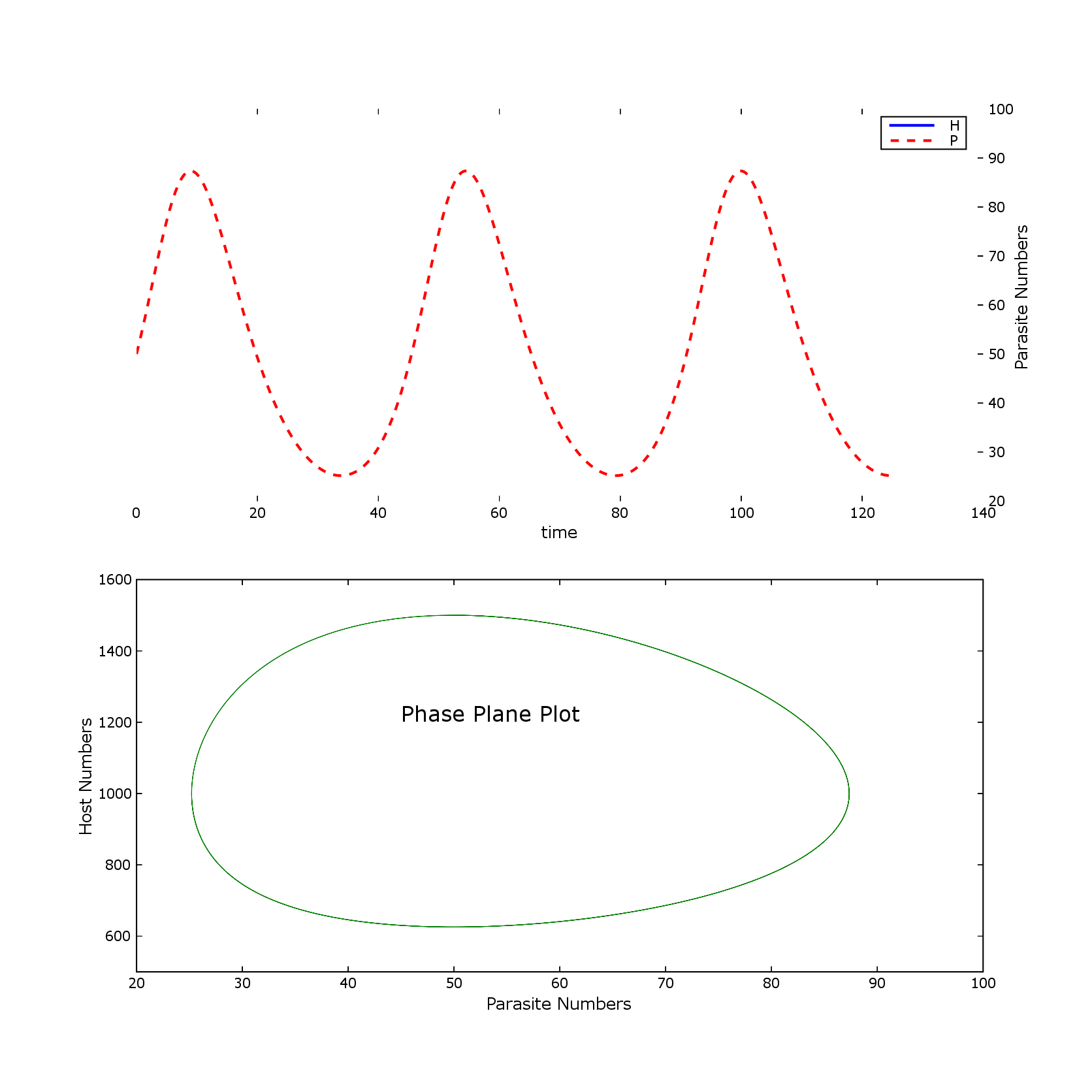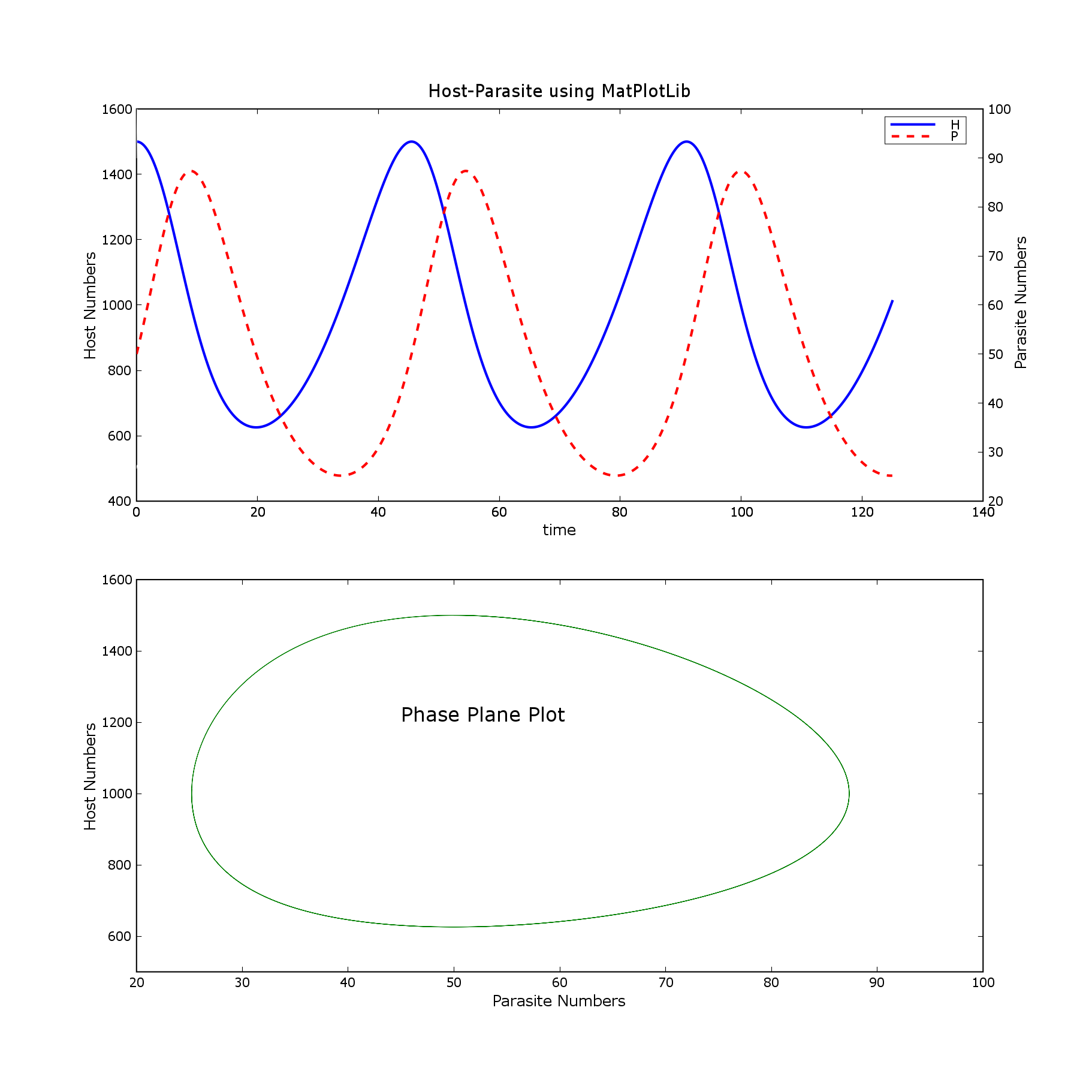# problem with multiaxis plot

'''Hi,

I use the following program in a simulation class to demonstrate the
niftyness of matplotlib. The combination of Python, Numeric, and matplotlib
really makes a great set of tools for biologists who are largely
non-programmers these days. Thanks.

I just did a big upgrade from 0.6-before pylab to 0.80. This is the only
tested program that didn't produce the same results before and after:

Attached are matlab and pylab png files.

Is there another way of doing this that gets back to the original output?

Also, is it just my imagination, or does 0.8 take longer to display graphs
than previous versions. I haven't done any formal timing, but the delay
seems longer.

Thanks,

Wendell Cropper '''

from pylab import *

r = 0.1
g = 0.002
h = 0.0002
m = 0.2

def derivs(x, t):

d1dt = r* x - g * x * x # host

d2dt = h * x * x - m * x #parasite

return (d1dt, d2dt)

dt = 0.01 #delta t

tim = arange(0.0, 125.0, dt)

x0 = (1500.0, 50.0) #initial numbers of host, parasite

xint = rk4(derivs, x0, tim) #Runge-Kutta 4th order numerical integration

#print xint
#print xint

host, parasite = [[x for x in xint], [x for x in xint]] #extract
variables

figure(1, [11.0, 11.0]) #size of window

ax1 = subplot(211)
title('Host-Parasite using MatPlotLib')
L1 = plot(tim, host, 'b', linewidth=2)
plot ([0.0, 0.1], [500.0, 510.0], 'w')
ax1.yaxis.tick_left()
ylabel('Host Numbers')

ax2 = subplot(211, frameon = False)
ax2.yaxis.tick_right()
text (145.0, 47.0, 'Parasite Numbers', rotation = 'vertical')
xlabel('time')
L2 = plot(tim, parasite, 'r--', linewidth=2)
plot ([0.0, 0.1], [90.0, 100.0], 'w')
legend((L1, L2), ('H', 'P'))

subplot(212)

plot(parasite, host, 'g')
axis([20.0, 100.0, 500.0, 1600.0]) #min x, max x, min y, max y
xlabel('Parasite Numbers')
ylabel('Host Numbers')
text (45.0, 1200.0, 'Phase Plane Plot', fontsize = 16)

show()